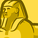# New to Qlik Sense

If you’re new to Qlik Sense, start with this Discussion Board and get up-to-speed quickly.

Announcements
cancel
Showing results for
Did you mean:Contributor

Hello everyone!

I have a table on my dashboard with values of orders and requests. And I want to compare these two values using backward color and a filter. My code with backward color works fine but I have problems with adding a filter pane. Now I have this:

=if(((([Order Value])-([Request Value]))/(([Request Value])))<=-0.3,'less than request on 30%',
if(((([Order Value])-([Request Value]))/(([Request Value])))<=-0.1,'less than request from 10 to 30%',
if(((([Order Value])-([Request Value]))/(([Request Value])))<=0.1,'don't differ from request more than 10%',
if(((([Order Value])-([Request Value]))/(([Request Value])))<=0.3,'more than request from 10% to 30%',
'more than request on 30%'))))

And when I use my filter to the table it shows me right values and some not the right ones. I can't understand why this is happening. Really need your help!

13 RepliesCreator III

If possible, post the sample app plsMVP

@Tatiana wrote:

And when I use my filter to the table it shows me right values and some not the right ones.

it shows the right values and what?Contributor
Author

As example: I use filter pane that should show me orders that are less than requests on 30% and more. I when I click this filter it shows me right cases and also cases when orders more than requests or equal to themMVP
Would you be able to share a sample file where we can see the issue?Contributor
Author
Can't attach QVF file to the message 😞MVP

Try to zip the file and then attach?Contributor
Author
done! thank youMVP

I suggest creating this in the script

```if((([Order]-[Request])
/([Request]))<=-0.3,'less than request 30%',
if((([Order]-[Request])
/(([Request])))<=-0.1,'less than request 10 to 30%',
if(((([Order])-([Request]))
/([Request]))<=0.1,'do not differ from request more than 10%',
if((([Order]-[Request])
/([Request]))<=0.3,'more than request 10% to 30%',
'more than request 30%'
)))) as NewFieldName```

and then use NewFieldName as your filter object....Contributor
Author
Tried, didn't work out. Can't understand what is the problem with this code